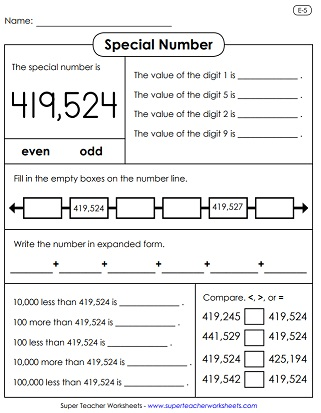# Place Value: 6 Digits (Hundred Thousands)

Welcome to the 6-digit place value page. Below, you'll find a large selection of printable games and worksheets to teach students about 6-digit place value. Topics include writing numbers in standard form, expanded notation, and word name format.## Place Value

Write the value for each of the given underlined digits.
Cut out the rectangles and organize them into groups of equivalent numbers. Also, classify each as standard form, word name, or expanded form.
Write the word name for each number; Up to 999,999
Students are given digits.  Students arrange the digits to make the numbers given by the teacher. They they answer questions about the numbers.
Practice place value to the hundred thousands place with this printable puzzle. Match the number with the correct expanded form.
Glue the name of each place over the correct digit in the number shown. (Ones, tens, hundreds, thousands, ten thousands, hundred thousands)
Students answer the place value questions; Up to hundred thousands place
Insert digits into the cells of this table to build 6-digit numbers.
Students rearrange the sets of 6 digits to make the smallest number and the largest number possible.
Match the word name with the standard form number; Numbers up to hundred thousands.
Students give the value of the underlined digit; Numbers up to hundred thousands.
Figure out the mystery numbers by putting the digits in the correct place; Place value up to hundred thousands.

## Special Numbers

On this worksheet, students will state the value of the digits 3, 9, 0, 2, and 4. They must also complete the numbers line. Then subtract 100,000, 1,000, and 100 from the special number.
This worksheet has a set of place value questions to go along with the number one hundred seventy-five thousand, twenty eight.
Complete the number line. Compare numbers. Tell whether the special number is odd or even. Write the values of the digits.
Compare the number 564,300 to other numbers using the symbols <, >, and =. Then tell if the number is odd or even. Write the number in expanded form. Give the values of the digits.
This page has a whole set of place value questions to go along with the number four hundred nineteen thousand, five hundred twenty-four.

## Ordering Numbers

Read the numbers in each box. Then rewrite them in the correct order, from the lowest to the highest.

## Comparing Numbers

Compare large numbers up to six digits.  Includes word problems, comparing with words, and using greater than / less than symbols.
On these place value task cards, students will use the <, >, and = symbols to compare numbers up to six digits.
The gators are gobbling up huge 6-digit numbers now.  Cut and glue the symbols in the correct place and write the answer.

Students read aloud the 6-digit numbers. Includes two grade sheets to send home.
Say the names of the 6-digit numbers.  Includes a homework sheet and a test.
Spin the number wheel and read the six-digit numbers aloud.
7-Digit Place Value

## Worksheet ImagesMy Account
Site Information